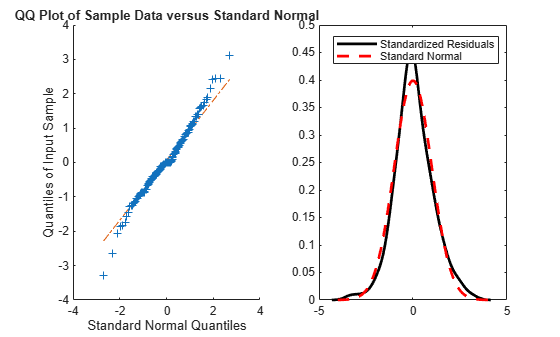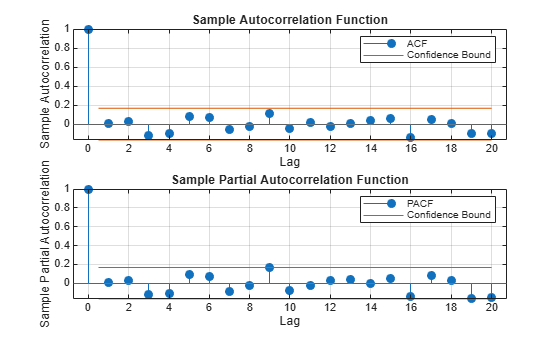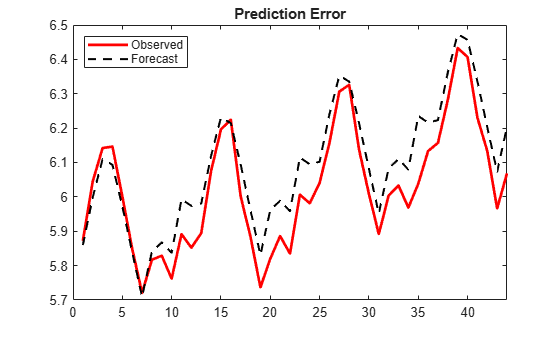# Check Fit of Multiplicative ARIMA Model

This example shows how to do goodness of fit checks. Residual diagnostic plots help verify model assumptions, and cross-validation prediction checks help assess predictive performance. The time series is monthly international airline passenger numbers from 1949 to 1960.

### Load the data and estimate a model.

```load Data_Airline y = log(Data); T = length(y); Mdl = arima('Constant',0,'D',1,'Seasonality',12,... 'MALags',1,'SMALags',12); EstMdl = estimate(Mdl,y);```
``` ARIMA(0,1,1) Model Seasonally Integrated with Seasonal MA(12) (Gaussian Distribution): Value StandardError TStatistic PValue _________ _____________ __________ __________ Constant 0 0 NaN NaN MA{1} -0.37716 0.066794 -5.6466 1.6364e-08 SMA{12} -0.57238 0.085439 -6.6992 2.0952e-11 Variance 0.0012634 0.00012395 10.193 2.1406e-24 ```

### Check the residuals for normality.

One assumption of the fitted model is that the innovations follow a Gaussian distribution. Infer the residuals, and check them for normality.

```res = infer(EstMdl,y); stres = res/sqrt(EstMdl.Variance); figure subplot(1,2,1) qqplot(stres) x = -4:.05:4; [f,xi] = ksdensity(stres); subplot(1,2,2) plot(xi,f,'k','LineWidth',2); hold on plot(x,normpdf(x),'r--','LineWidth',2) legend('Standardized Residuals','Standard Normal') hold off```The quantile-quantile plot (QQ-plot) and kernel density estimate show no obvious violations of the normality assumption.

### Check the residuals for autocorrelation.

Confirm that the residuals are uncorrelated. Look at the sample autocorrelation function (ACF) and partial autocorrelation function (PACF) plots for the standardized residuals.

```figure subplot(2,1,1) autocorr(stres) subplot(2,1,2) parcorr(stres)````[h,p] = lbqtest(stres,'lags',[5,10,15],'dof',[3,8,13])`
```h = 1x3 logical array 0 0 0 ```
```p = 1×3 0.1842 0.3835 0.7321 ```

The sample ACF and PACF plots show no significant autocorrelation. More formally, conduct a Ljung-Box Q-test at lags 5, 10, and 15, with degrees of freedom 3, 8, and 13, respectively. The degrees of freedom account for the two estimated moving average coefficients.

The Ljung-Box Q-test confirms the sample ACF and PACF results. The null hypothesis that all autocorrelations are jointly equal to zero up to the tested lag is not rejected (`h = 0`) for any of the three lags.

### Check predictive performance.

Use a holdout sample to compute the predictive MSE of the model. Use the first 100 observations to estimate the model, and then forecast the next 44 periods.

```y1 = y(1:100); y2 = y(101:end); Mdl1 = estimate(Mdl,y1);```
``` ARIMA(0,1,1) Model Seasonally Integrated with Seasonal MA(12) (Gaussian Distribution): Value StandardError TStatistic PValue _________ _____________ __________ __________ Constant 0 0 NaN NaN MA{1} -0.35674 0.089461 -3.9876 6.6739e-05 SMA{12} -0.63319 0.098744 -6.4124 1.4326e-10 Variance 0.0013285 0.00015882 8.365 6.013e-17 ```
```yF1 = forecast(Mdl1,44,'Y0',y1); pmse = mean((y2-yF1).^2)```
```pmse = 0.0069 ```
```figure plot(y2,'r','LineWidth',2) hold on plot(yF1,'k--','LineWidth',1.5) xlim([0,44]) title('Prediction Error') legend('Observed','Forecast','Location','northwest') hold off```The predictive ability of the model is quite good. You can optionally compare the PMSE for this model with the PMSE for a competing model to help with model selection.